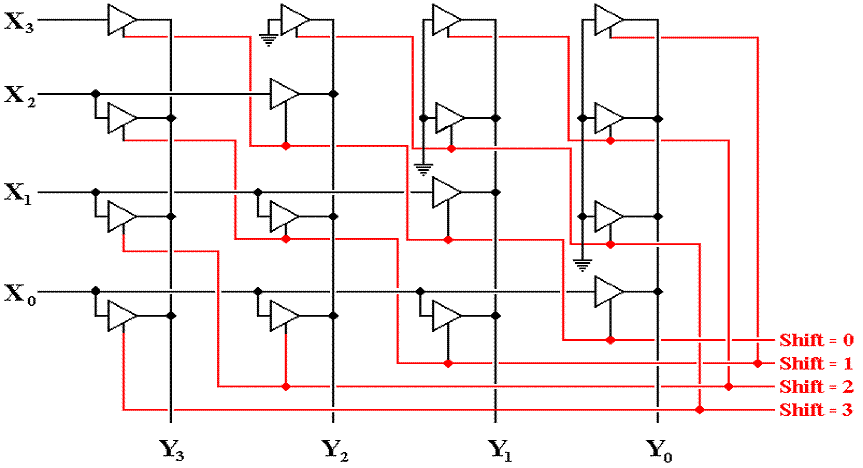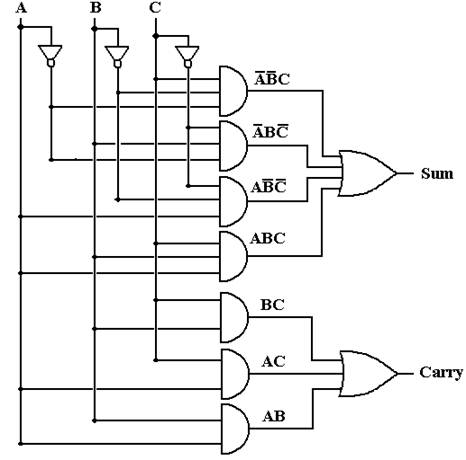This basic circuit hides the dynamics associated with changing values.

Gate Delays

Consider three gates, each of which has input that has not changed for
some time.  The output of each gate correctly implements its Boolean function.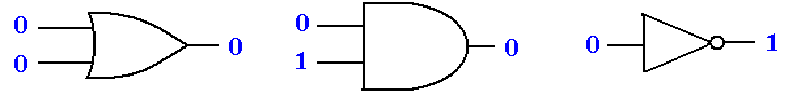We now consider what happens as the input to each gate is changed
suddenly.  Note that the output does not change at the same time as the input.

For a short time, called a “hazard”, the output of the gate is not that
of the Boolean function supposedly implemented.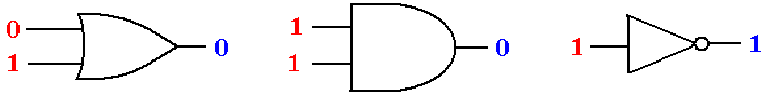Gate Delays (More)

For the time duration of one gate delay, the outputs do not match the inputs.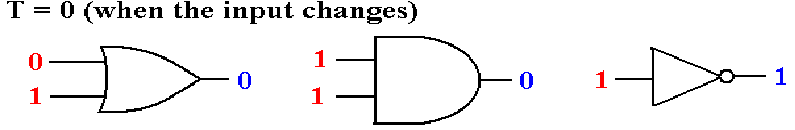After one gate delay (about 2 to 10 nanoseconds) the output is correct.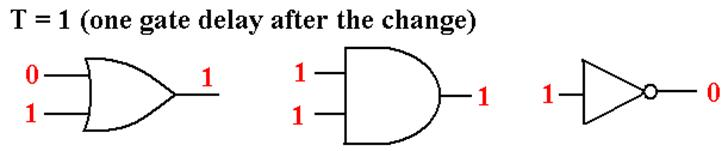Full Adder with Gate Delays (Before Input Changes)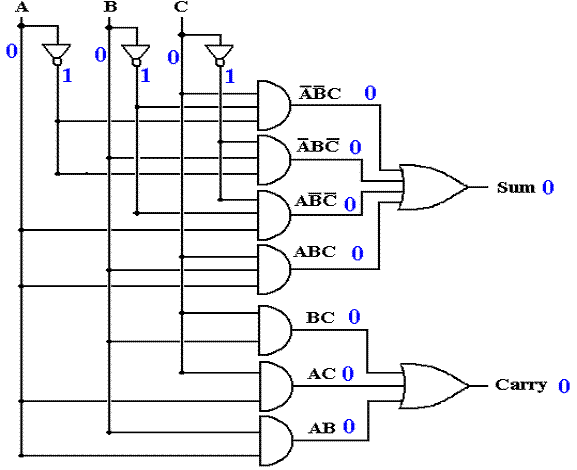Assume the inputs have been stable for some time.

Full Adder with Gate Delays (Input Changes at T = 0)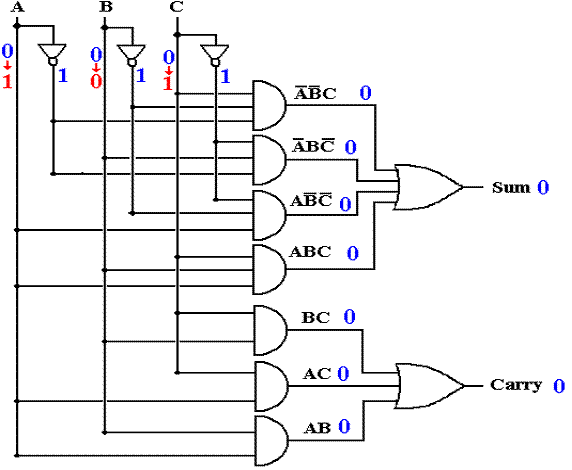At this point, no outputs are correct.

Full Adder with Gate Delays (T = 1)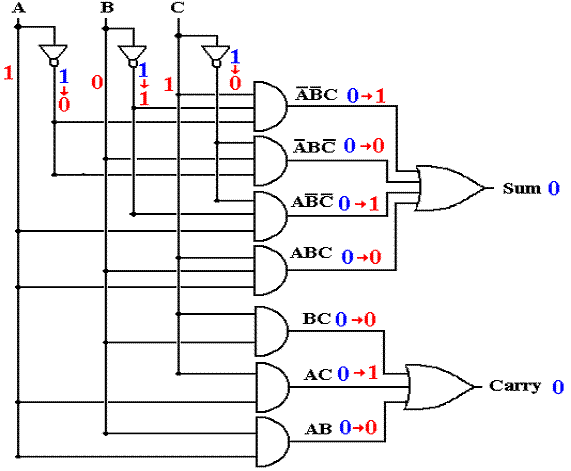Some output is correct, but the upper AND gates are reacting to input at T = 0.

Full Adder with Gate Delays (T = 2)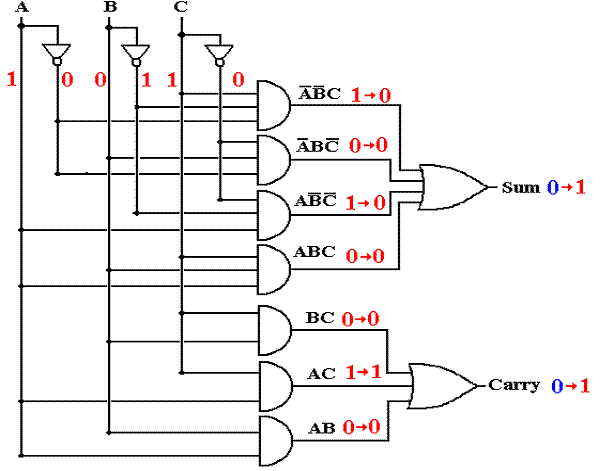The carry out is correct, but the sum out is reacting to input at T = 1.

Full Adder with Gate Delays (T = 3)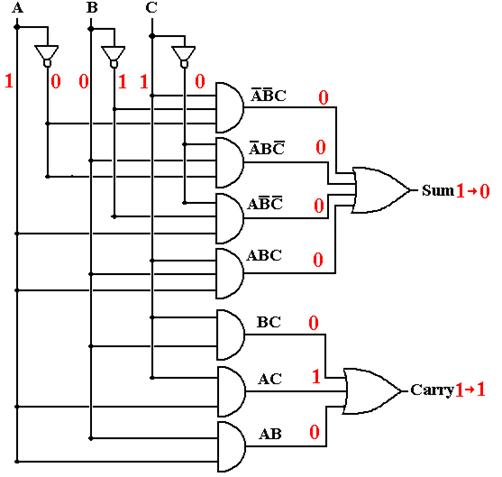At T = 3 (and later), all output is correct.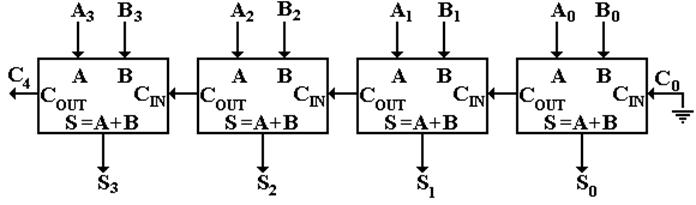Carry–in to stage K (bit K) is valid at T = 2·K.

Carry–out from stage K is valid at T = 2·K + 2.

Sum from stage K is valid at T = 2·K + 3.

32–bit sum valid after stage 31 finishes (65 gate delays)
This circuit is not used in commercial computers.

Shifters

Logical Shifts
Left Shift                                    Right Shift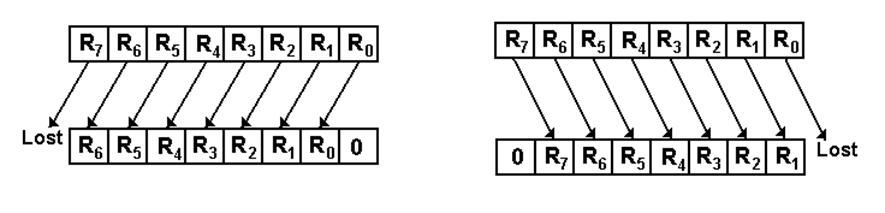Arithmetic Shifts (Preserve the Sign Bit)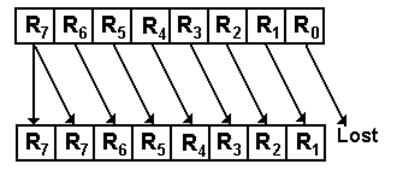Shifters (Continued)

Circular Shifts
Left Circular                                           Right Circular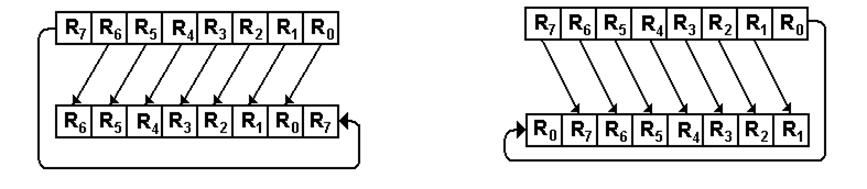No bits are lost.

Circuit for Logical Left Shift
(Only four bits shown)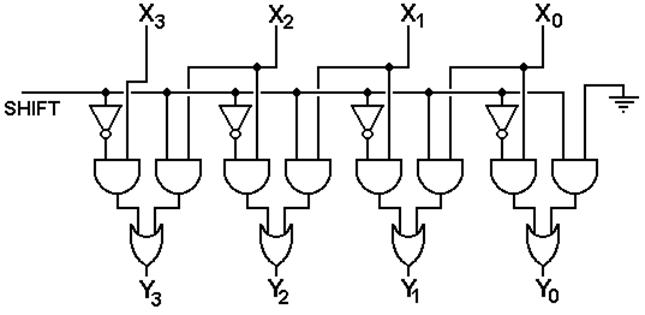X is the input and Y is the output

Circuit for Logical Left Shift
(Simpler Notation)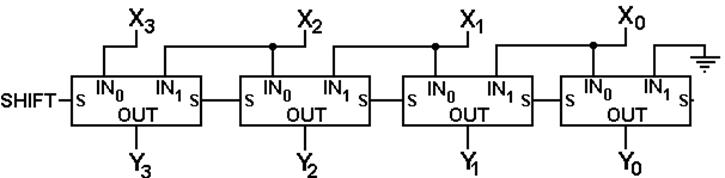Two-Stage Logical Left Shifter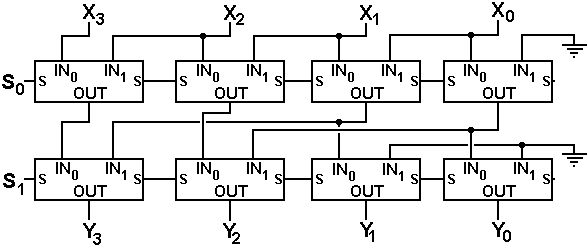The binary number S1S0 indicates the size of the shift.

Two Stage Left Shifter with Logical and Circular Shifts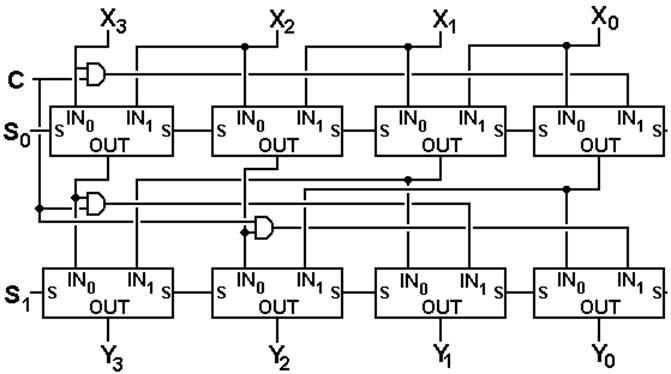C = 0 for logical shift
C = 1 for circular shift

S1S0 indicates the size of the shift.

Thirty–Two Bit Shifter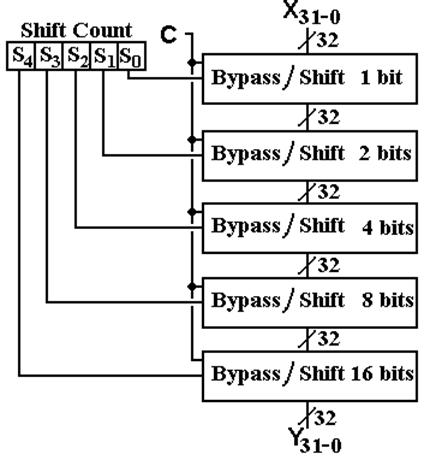This is a “logarithmic shifter”.  A cross–bar shifter is much more complex.

Cross–Bar Shifters

Cross–bar shifters can perform any number of shifts in one stage.
However, they are much more complex to draw.

Cross–bar shifters require either tri–state buffers or AND/OR gate
combinations to attach each input to an output.

For N bits, there are N input lines and N output lines.

Thus an N–bit cross–bar shifter requires at least N2 gates.

An N–bit cross–bar shifter may be implemented with N2 tri–state buffers.

Each input line may be potentially connected to any one of the N output
lines, thus there are N tri–state buffers for each input line.

If N = 4, the tri–state count is 16

If N = 32, the tri–state count is 4,096.

Cross–Bar Shifters for Circular Shifts

Here is a four–bit cross–bar shifter for circular left shifts.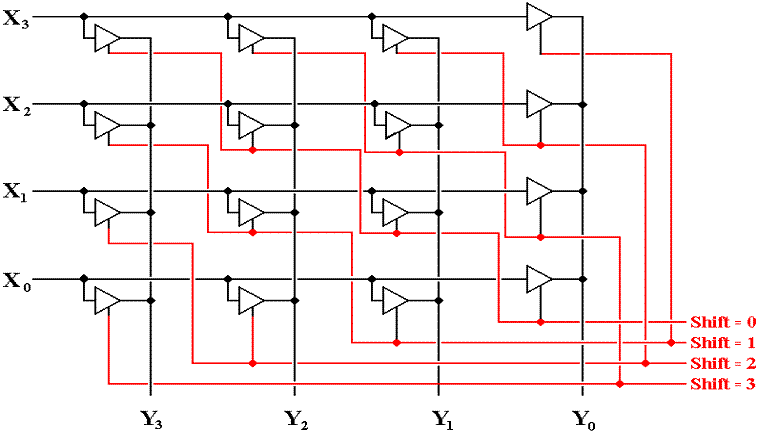Cross–Bar Shifters for Logical Shifts

Here is a four–bit cross–bar shifter for circular left shifts.